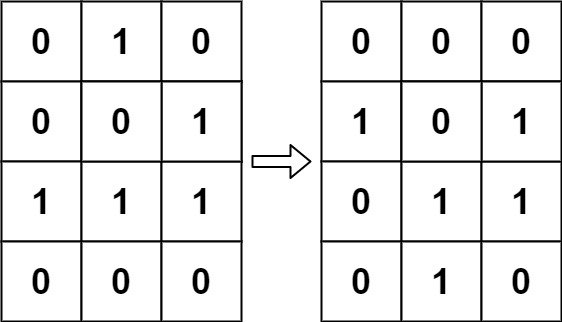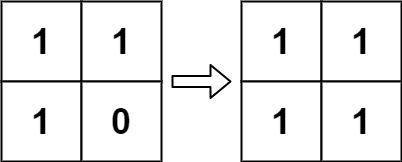## Algorithm

Problem Name: 289. Game of Life

According to Wikipedia's article: "The Game of Life, also known simply as Life, is a cellular automaton devised by the British mathematician John Horton Conway in 1970."

The board is made up of an `m x n` grid of cells, where each cell has an initial state: live (represented by a `1`) or dead (represented by a `0`). Each cell interacts with its eight neighbors (horizontal, vertical, diagonal) using the following four rules (taken from the above Wikipedia article):

1. Any live cell with fewer than two live neighbors dies as if caused by under-population.
2. Any live cell with two or three live neighbors lives on to the next generation.
3. Any live cell with more than three live neighbors dies, as if by over-population.
4. Any dead cell with exactly three live neighbors becomes a live cell, as if by reproduction.

The next state is created by applying the above rules simultaneously to every cell in the current state, where births and deaths occur simultaneously. Given the current state of the `m x n` grid `board`, return the next state.

Example 1:```Input: board = [[0,1,0],[0,0,1],[1,1,1],[0,0,0]]
Output: [[0,0,0],[1,0,1],[0,1,1],[0,1,0]]
```

Example 2:```Input: board = [[1,1],[1,0]]
Output: [[1,1],[1,1]]
```

Constraints:

• `m == board.length`
• `n == board[i].length`
• `1 <= m, n <= 25`
• `board[i][j]` is `0` or `1`.

## Code Examples

### #1 Code Example with C Programming

```Code - C Programming```

``````
void update(int **board, int rowsz, int colsz, int *visited, int x, int y) {
int a, b, c, d, e, f, g, h;

int t;

if (x < 0 || x >= rowsz ||
y < 0 || y >= colsz ||
visited[x * colsz + y]) return;

visited[x * colsz + y] = 1;

t = 0;

if (x > 0) {
t +=  board[x - 1][y];
}
if (x > 0 && y < colsz - 1) {
t += board[x - 1][y + 1];
}
if (y < colsz - 1) {
t += board[x][y + 1];
}
if (x < rowsz - 1 && y < colsz - 1) {
t += board[x + 1][y + 1];
}
if (x < rowsz - 1) {
t += board[x + 1][y];
}
if (x < rowsz - 1 && y > 0) {
t += board[x + 1][y - 1];
}
if (y > 0) {
t += board[x][y - 1];
}
if (x > 0 && y > 0) {
t += board[x - 1][y - 1];
}

update(board, rowsz, colsz, visited, x - 1, y);
update(board, rowsz, colsz, visited, x - 1, y + 1);
update(board, rowsz, colsz, visited, x, y + 1);
update(board, rowsz, colsz, visited, x + 1, y + 1);
update(board, rowsz, colsz, visited, x + 1, y);
update(board, rowsz, colsz, visited, x + 1, y - 1);
update(board, rowsz, colsz, visited, x, y - 1);
update(board, rowsz, colsz, visited, x - 1, y - 1);

if (board[x][y]) {
if (t < 2 || t > 3) board[x][y] = 0;
} else {
if (t == 3) board[x][y] = 1;
}
}
void gameOfLife(int** board, int boardRowSize, int boardColSize) {
int *visited = calloc(boardRowSize * boardColSize, sizeof(int));
//assert(visited);
update(board, boardRowSize, boardColSize, visited, 0, 0);
free(visited);
}
``````
Copy The Code &

Input

cmd
board = [[0,1,0],[0,0,1],[1,1,1],[0,0,0]]

Output

cmd
[[0,0,0],[1,0,1],[0,1,1],[0,1,0]]

### #2 Code Example with C++ Programming

```Code - C++ Programming```

``````
class Solution {
public:
void gameOfLife(vector>& board) {
int m = board.size() - 1, n = board.size() - 1;
for(int i = 0; i <= m; i++)
for(int j = 0; j <= n; j++){
int neigh = cntNeighbor(board, m, n, i, j), cur = board[i][j];
board[i][j] = (neigh > 3 || neigh < 2) && cur ? 2 : (neigh == 3 && !cur) ? -1 : cur;
}

for(auto& x: board)
for(auto& y: x)
y = (y == 2) ? 0 : (y == -1) ? 1 : y;
}

int cntNeighbor(vector>& board, int m, int n,  int r, int c){
int cnt = 0;
for(int i = max(0, r - 1); i <= min(m, r + 1); i++)
for(int j = max(0, c - 1); j <= min(n, c + 1); j++)
if((i != r || j != c) && board[i][j] > 0) cnt++;
return cnt;
}
};
``````
Copy The Code &

Input

cmd
board = [[0,1,0],[0,0,1],[1,1,1],[0,0,0]]

Output

cmd
[[0,0,0],[1,0,1],[0,1,1],[0,1,0]]

### #3 Code Example with Java Programming

```Code - Java Programming```

``````
class Solution {

private static final int[][] DIRS = {{1, 0}, {0, 1}, {-1, 0}, {0, -1}, {1, 1}, {1, -1}, {-1, 1}, {-1, -1}};

public void gameOfLife(int[][] board) {
int rows = board.length;
int cols = board.length;
for (int i = 0; i < rows; i++) {
for (int j = 0; j < cols; j++) {
int liveNeighborCount = getLiveNeighbours(board, i, j);
if (board[i][j] == 1) {
if (liveNeighborCount < 2 || liveNeighborCount > 3) {
board[i][j] = 2;
}
} else {
if (liveNeighborCount == 3) {
board[i][j] = 3;
}
}
}
}
for (int i = 0; i < rows; i++) {
for (int j = 0; j < cols; j++) {
if (board[i][j] == 2) {
board[i][j] = 0;
} else if (board[i][j] == 3) {
board[i][j] = 1;
}
}
}
}

private static int getLiveNeighbours(int[][] board, int i, int j) {
int count = 0;
for (int[] dir : DIRS) {
int x = i + dir;
int y = j + dir;
if (isValidCell(board, x, y) && (board[x][y] == 1 || board[x][y] == 2)) {
count++;
}
}
return count;
}

private static boolean isValidCell(int[][] board, int i, int j) {
return i >= 0 && j >= 0 && i < board.length && j < board.length;
}
}
``````
Copy The Code &

Input

cmd
board = [[1,1],[1,0]]

Output

cmd
[[1,1],[1,1]]

### #4 Code Example with Javascript Programming

```Code - Javascript Programming```

``````
const gameOfLife = function(board) {
const DIRECTIONS = [
[1, 0],
[1, 1],
[0, 1],
[-1, 1],
[-1, 0],
[-1, -1],
[0, -1],
[1, -1]
];

const isValid = function(x, y) {
if (x >= 0 && y >= 0 && x < board.length && y < board.length)
return true;
else return false;
};

const getAliveNeighbors = function(x, y) {
let aliveNeighs = 0;
for (let dir of DIRECTIONS) {
let newX = x + dir;
let newY = y + dir;
if (!isValid(newX, newY)) continue;
if (board[newX][newY] === 1 || board[newX][newY] === -1) {
aliveNeighs++;
}
}

return aliveNeighs;
};

for (let row = 0; row < board.length; row++) {
for (let col = 0; col < board.length; col++) {
let aliveNeighbors = getAliveNeighbors(row, col);
if (board[row][col] === 0) {
if (aliveNeighbors === 3) board[row][col] = 2;
else board[row][col] = 0;
} else {
if (aliveNeighbors === 2 || aliveNeighbors === 3) board[row][col] = 1;
else board[row][col] = -1;
}
}
}

for (let row = 0; row < board.length; row++) {
for (let col = 0; col < board.length; col++) {
if (board[row][col] > 0) board[row][col] = 1;
else board[row][col] = 0;
}
}
};
``````
Copy The Code &

Input

cmd
board = [[1,1],[1,0]]

Output

cmd
[[1,1],[1,1]]

### #5 Code Example with Python Programming

```Code - Python Programming```

``````
class Solution:
def gameOfLife(self, board):
m, n = len(board), len(board)
matrix = [ * n for i in range(m)]
for i in range(m):
for j in range(n):
cnt = 0
for x, y in ((i - 1, j), (i + 1, j), (i, j - 1), (i, j + 1), (i - 1, j - 1), (i + 1, j + 1), (i - 1, j + 1), (i + 1, j - 1)):
if 0 <= x < m and 0 <= y < n and board[x][y] == 1: cnt += 1
if (board[i][j] and 2 <= cnt <= 3) or (not board[i][j] and cnt == 3): matrix[i][j] = 1
for i in range(m):
for j in range(n):
board[i][j] = matrix[i][j]
``````
Copy The Code &

Input

cmd
board = [[0,1,0],[0,0,1],[1,1,1],[0,0,0]]

Output

cmd
[[0,0,0],[1,0,1],[0,1,1],[0,1,0]]

### #6 Code Example with C# Programming

```Code - C# Programming```

``````
using System;

namespace LeetCode
{
public class _0289_GameOfLife
{
public void GameOfLife(int[][] board)
{
int[] neighbors = { 0, 1, -1 };

int rowsCount = board.Length;
int colsCount = board.Length;

for (int row = 0; row < rowsCount; row++)
for (int col = 0; col < colsCount; col++)
{
int liveNeighbors = 0;

for (int i = 0; i < 3; i++)
for (int j = 0; j < 3; j++)
if (!(neighbors[i] == 0 && neighbors[j] == 0))
{
int r = (row + neighbors[i]);
int c = (col + neighbors[j]);

if ((r < rowsCount && r >= 0) && (c < colsCount && c >= 0) && (Math.Abs(board[r][c]) == 1))
liveNeighbors += 1;
}

if ((board[row][col] == 1) && (liveNeighbors < 2 || liveNeighbors > 3))
board[row][col] = -1;
if (board[row][col] == 0 && liveNeighbors == 3)
board[row][col] = 2;
}

for (int row = 0; row < rowsCount; row++)
for (int col = 0; col < colsCount; col++)
if (board[row][col] > 0)
board[row][col] = 1;
else
board[row][col] = 0;
}
}
}
``````
Copy The Code &

Input

cmd
board = [[0,1,0],[0,0,1],[1,1,1],[0,0,0]]

Output

cmd
[[0,0,0],[1,0,1],[0,1,1],[0,1,0]]